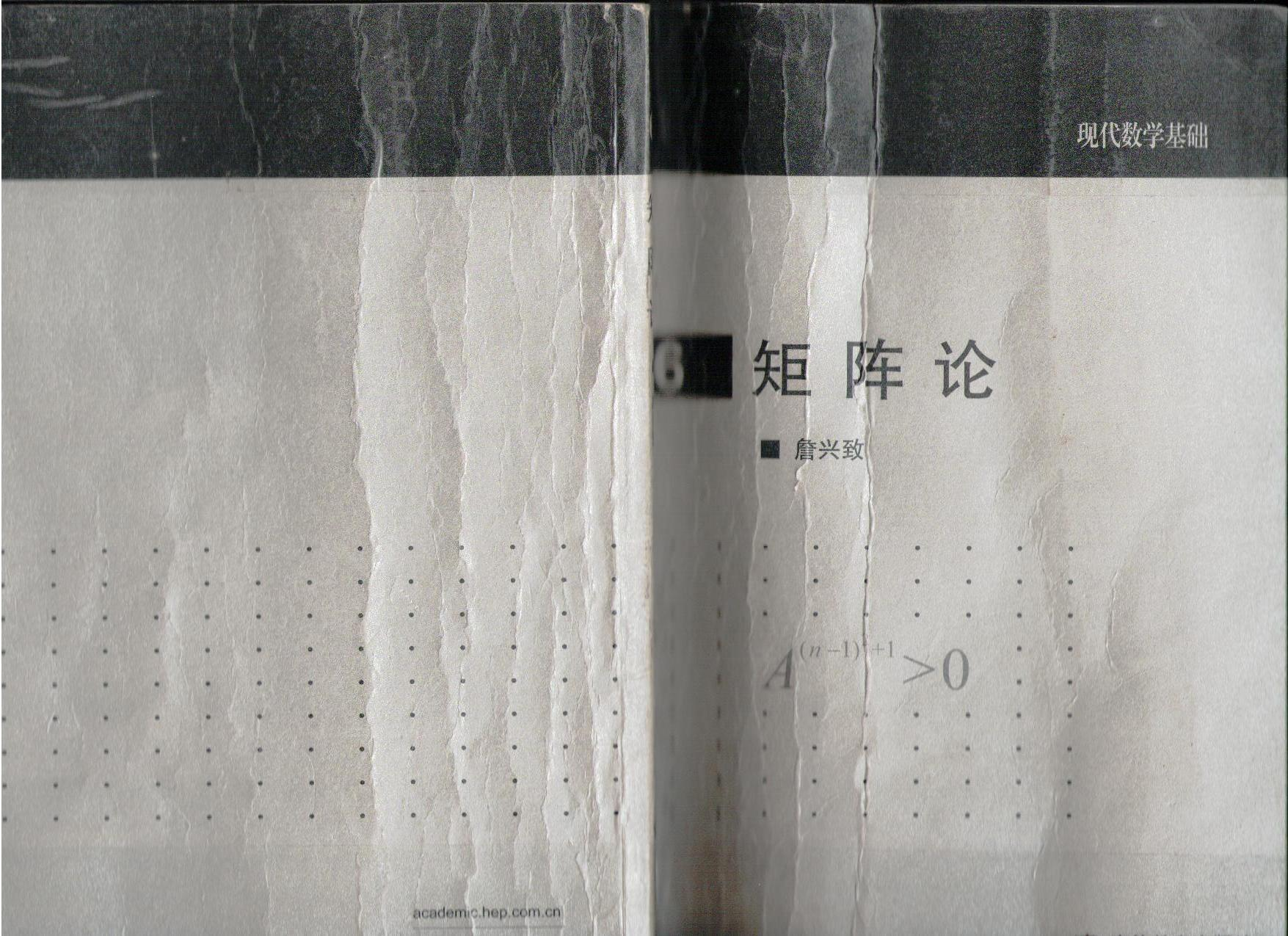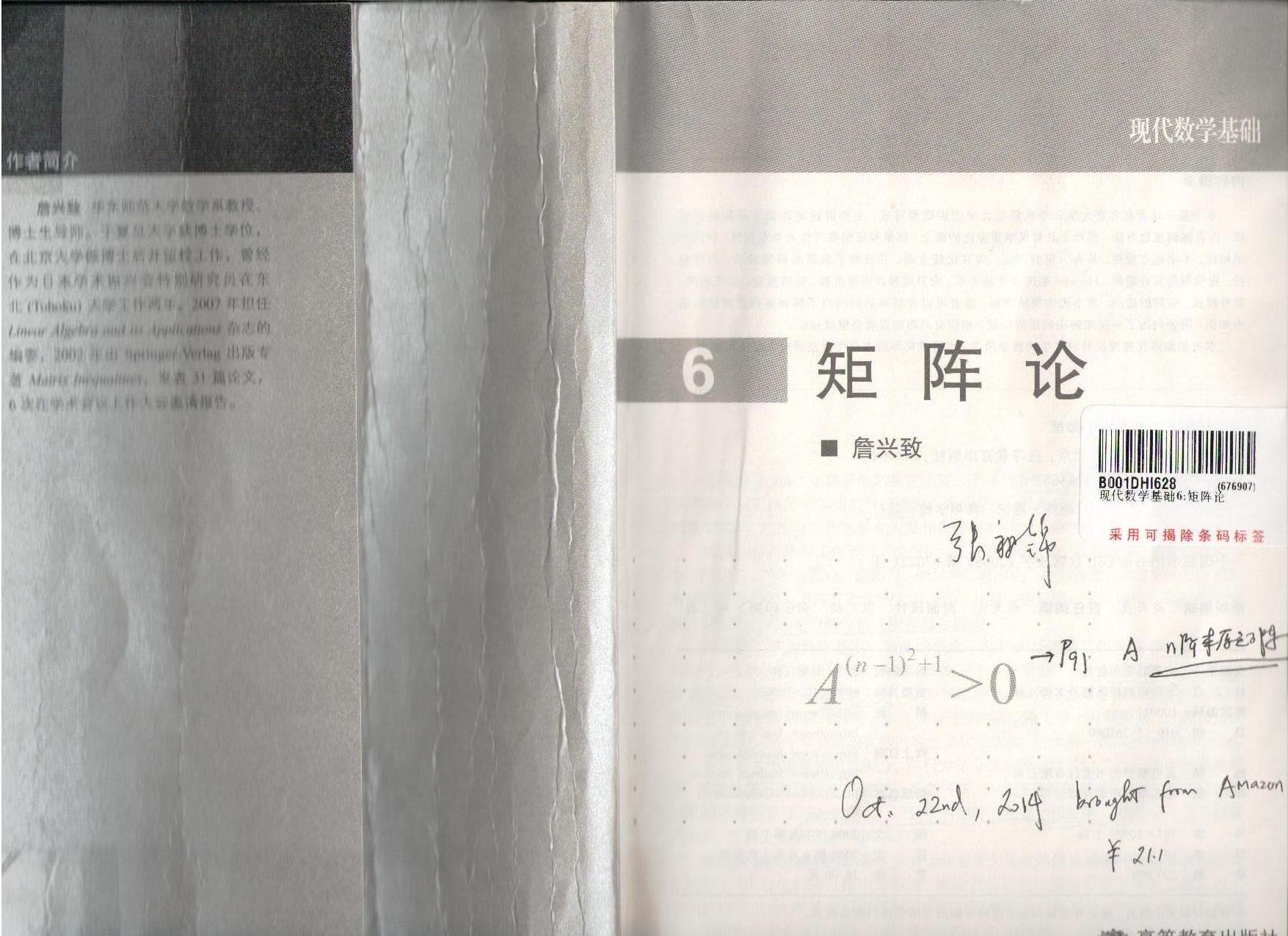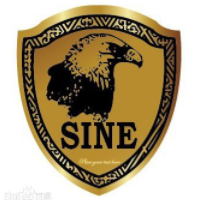[家里蹲大学数学杂志]第328期詹兴致矩阵论习题参考解答

+关注继续查看1. 大部分是自己做的, 少部分是参考文献做的, 还有几个直接给出参考文献.

2. 如果您有啥好的想法, 好的解答, 热切地欢迎您告知我, 或者在相应的习题解答网页上回复. 哪里有错误, 也盼望您指出.

3. 毕竟大学时学过高等代数, 想多学点矩阵论的东西 (matrix=magic), 就先选这本书看看了.

目录

[詹兴致矩阵论习题参考解答]习题1.1

1. 设 $a_1,\cdots,a_n$ 为正实数, 证明矩阵 $$\bex \sex{\frac{1}{a_i+a_j}}_{n\times n} \eex$$ 半正定.

[詹兴致矩阵论习题参考解答]习题1.2

2. (Oldenburgere) 设 $A\in M_n$, $\rho(A)$ 表示 $A$ 的谱半径, 即 $A$ 的特征值的模的最大者. 证明: $$\bex \vlm{k}A^k=0\lra \rho(A)<1. \eex$$

[詹兴致矩阵论习题参考解答]习题1.3

3. 证明数值半径 $w(\cdot)$ 是 $M_n$ 上的一个范数.

[詹兴致矩阵论习题参考解答]习题1.4

4. 证明数值半径 $w(\cdot)$ 和谱范数 $\sen{\cdot}_\infty$ 满足如下关系: $$\bex \frac{1}{2}\sen{A}_{\infty} \leq w(A)\leq \sen{A}_\infty,\quad A\in M_n. \eex$$

[詹兴致矩阵论习题参考解答]习题1.5

5. (Gelfand) 设 $A\in M_n$, 证明: $$\bex \rho(A)=\vlm{k}\sen{A^k}_\infty^\frac{1}{k}. \eex$$

[詹兴致矩阵论习题参考解答]习题1.6

6. 设 $A\in M_{m,n}$, $B\in M_{n,m}$. 证明: $$\bex \sex{\ba{cc} AB&0\\ B&0 \ea}\mbox{ 和 }\sex{\ba{cc} 0&0\\ B&BA \ea} \eex$$ 相似, 从而给出定理 1.14 的另一个证明.

[詹兴致矩阵论习题参考解答]习题1.7

7. 设 $A_j\in M_n$, $j=1,\cdots,m$, $m>n$, 且 $\dps{\sum_{j=1}^m A_j}$ 非奇异 (即可逆). 证明: 存在 $S\subset \sed{1,2,\cdots,m}$ 满足 $|S|\leq n$ 且 $\dps{\sum_{j\in S}A_j}$ 非奇异.

[詹兴致矩阵论习题参考解答]习题1.8

8. 证明任何一个复方阵都酉相似于某个对角元素全部相等的矩阵.

9. 证明对任意的复方阵 $A$, $$\bex \rho(A)\leq w(A)\leq \sen{A}_\infty. \eex$$

[詹兴致矩阵论习题参考解答]习题1.10

10. 矩阵 $A=(a_{ij})\in M_n$ 称为严格对角占优, 如果 $$\bex |a_{ii}|>\sum_{j\neq i}|a_{ij}|,\quad i=1,\cdots,n. \eex$$ 证明: 严格对角占优矩阵是可逆的.

[詹兴致矩阵论习题参考解答]习题1.11

11.  (Gersgorin 圆盘定理) 用 $\sigma(A)$ 表示 $A=(a_{ij})\in M_n$ 的特征值的集合, 记 $$\bex D_i=\sed{z\in\bbC;\ |z-a_{ii}|\leq \sum_{j\neq i}|a_{ij}|},\quad i=1,\cdots,n. \eex$$ 证明: $$\bex \sigma(A)\subset \cup_{i=1}^n D_i, \eex$$ 并且如果这些圆盘 $D_i$ 中有 $k$ 个与其余的 $n-k$ 个不相交, 则这 $k$ 个圆盘的并集恰好含有 $A$ 的 $k$ 个特征值.

[詹兴致矩阵论习题参考解答]习题1.12

12. (Sherman-Morrison-Woodbury 公式) 设 $A\in M_n$, $B,C\in M_{n,k}$ 使得 $I+C^*A^{-1}B$ 可逆, 其中 $I$ 是单位阵. 证明 $A+BC^*$ 可逆且 $$\bex (A+BC^*)^{-1} =A^{-1} -A^{-1}B (I+C^*A^{-1}B)^{-1}C^*A^{-1}. \eex$$

[詹兴致矩阵论习题参考解答]习题1.13

13. (Li-Poon) 证明: 每个实方阵都可以写成 $4$ 个实正交矩阵的线性组合, 即若 $A$ 是个实方阵, 则存在实正交矩阵 $Q_i$ 和实数 $r_i$, $i=1,2,3,4$, 使得 $$\bex A=r_1Q_1+r_2Q_2+r_3Q_3+r_4Q_4. \eex$$

[詹兴致矩阵论习题参考解答]习题1.14

14. 如果映射 $f:M_n\to M_n$ 按某个固定的模式将 $M_n$ 中的每个矩阵的元素重排, 则称 $f$ 为一个置换算子. 怎样的置换算子保持矩阵的特征值不变? 保持秩不变?

[詹兴致矩阵论习题参考解答]习题2.1

1. 对于怎样的 $A\in M_m$, $B\in M_n$, $A\otimes B=I$?

[詹兴致矩阵论习题参考解答]习题2.2

2. 给出定理 2.4 的另一个证明.

[詹兴致矩阵论习题参考解答]习题2.3

3. 设 $A,B\in M_n$, $A$ 正定, $B$ 半正定且对角元素都是正数, 则 $A\circ B$ 正定.

[詹兴致矩阵论习题参考解答]习题2.4

4. 设 $A=\diag(A_1,\cdots,A_k)\in M_n$, 其中 $A_i\in M_{n_i}$, 且 $\sigma(A_i)\cap \sigma(A_j)=\vno$, $i\neq j$. 若 $B\in M_n$ 且 $AB=BA$, 则 $B=\diag(B_1,\cdots,B_k)\in M_n$, 其中 $B_i\in M_{n_i}$.

[詹兴致矩阵论习题参考解答]习题2.5

5. 设 $A\in M_m$, $B\in M_n$, $C\in M_{m,n}$. 若 $\sigma(A)\cap \sigma(B)=\vno$, 则 $$\bex \sex{\ba{cc} A&C\\ 0&B \ea}\mbox{ 和 }\sex{\ba{cc} A&0\\ 0&B \ea}\mbox{ 相似}. \eex$$

[詹兴致矩阵论习题参考解答]习题2.6

6. (Embry) 我们说两个矩阵 $X$, $Y$ 可交换是指乘法可交换, 即 $XY=YX$. 设 $A,B\in M_n$ 满足 $\sigma(A)\cap \sigma(B)=\vno$. 如果 $C\in M_n$, $C$ 与 $A+B$ 可交换并且 $C$ 与 $AB$ 可交换, 则 $C$ 与 $A$ 和 $B$ 都可交换.

[詹兴致矩阵论习题参考解答]习题2.7

7. (Marcus-Ree) 一个非负矩阵称为是双随机的, 若它的每行元素之和等于 $1$, 且它的每列元素之和也等于 $1$. 设 $A=(a_{ij})$ 为 $n$ 阶双随机矩阵, 则存在 $1,2,\cdots,n$ 的一个排列 $\sigma$ 使得对每个 $i=1,\cdots,n$, $$\bex a_{i\sigma(i)}\geq \sedd{\ba{ll} \cfrac{1}{k(k+1)},&n=2k,\\ \cfrac{1}{(k+1)^2},&n=2k+1. \ea} \eex$$

[詹兴致矩阵论习题参考解答]习题2.8

8. 设 $k\leq m\leq n$. 怎样的矩阵 $A\in M_{m,n}$ 的每条对角线恰好含有 $k$ 个零元素?

[詹兴致矩阵论习题参考解答]习题2.9

9. 记 $\dps{m=\sex{n\atop k}}$. 复合矩阵映射 $C_k(\cdot): M_n\to M_m$ 是单射吗? 是满射吗?

[詹兴致矩阵论习题参考解答]习题3.1

1. 设 $A\in M_n$. 证明若 $AA^*=A^2$, 则 $A^*=A$.

[詹兴致矩阵论习题参考解答]习题3.2

2. 设 $A\in M_n$, $B\in M_{r,t}$ 是 $A$ 的一个子矩阵. 则它们的奇异值满足 $$\bex s_j(B)\leq s_j(A),\quad j=1,\cdots,\min\sed{r,t}. \eex$$

[詹兴致矩阵论习题参考解答]习题3.3

3. (Aronszajn) 设 $$\bex C=\sex{\ba{cc} A&X\\ X^*&B \ea} \eex$$ 为 Hermite 矩阵, $C\in M_n$, $A\in M_k$. 设 $A,B,C$ 的特征值分别为 $\al_1\geq \cdots\geq \al_k$, $\beta_1\geq \cdots \beta_{n-k}$, $\gamma_1\geq \cdots\geq \gamma_n$. 则对于满足 $i+j-1\leq n$ 的任意的 $i,j$, $$\bex \gamma_{i+j-1}+\gamma_n\leq \al_i+\beta_j. \eex$$

[詹兴致矩阵论习题参考解答]习题3.4

4. 设 $x,y,u\in\bbR^n$ 的分量都是递减的. 证明:

(1). 若 $x\prec y$ 则 $\sef{x,u}\leq \sef{y,u}$.

(2). 若 $x\prec_w y$ 且 $u\in\bbR^n_+$, 则 $\sef{x,u}\leq \sef{y,u}$.

[詹兴致矩阵论习题参考解答]习题3.5

5. 不用 Weierstrass 定理, 直接证明 Hermite 矩阵的函数运算 (3.6) 与特定的谱分解无关.

[詹兴致矩阵论习题参考解答]习题3.6

6. 设 $A,B\in M_n$, $A$ 是正定矩阵, $B$ 是 Hermite 矩阵. 则 $$\bex A+B\mbox{ 正定当且仅当 }\lm_j(A^{-1}B)>-1,\quad j=1,\cdots,n. \eex$$

[詹兴致矩阵论习题参考解答]习题3.7

7. 设 $A\in M_n$ 正定, $1\leq k\leq n$. 则 $$\bex \prod_{j=1}^n \lm_j(A)=\max_{U^*U=I_k} \det U^*AU,\quad \prod_{j=1}^n \lm_{n-j+1}(A)=\min_{U^*U=I_k} \det U^*AU, \eex$$ 其中 $U\in M_{n,k}$.

[詹兴致矩阵论习题参考解答]习题3.8

8. 证明每个半正定矩阵都有唯一的半正定平方根, 即若 $A\geq 0$, 则存在唯一的 $B\geq 0$ 满足 $B^2=A$.

[詹兴致矩阵论习题参考解答]习题3.9

9. 用公式 $$\bex t^r=\frac{\sin r\pi}{\pi}\int_0^\infty \frac{s^{r-1}t}{s+t}\rd s\quad \sex{0<r<1} \eex$$ 证明定理 3.24.

[詹兴致矩阵论习题参考解答]习题3.10

10. 设 $A,B$ 是同阶半正定矩阵, $0\leq s\leq 1$. 证明: $$\bex \sen{A^sB^s}_\infty \leq \sen{AB}_\infty^s. \eex$$

[詹兴致矩阵论习题参考解答]习题3.11

11. (Ky Fan) 对于 $A\in M_n$, 记 $\Re A=(A+A^*)/2$. 证明: $$\bex \Re \lm(A)\prec \lm(\Re A), \eex$$ 其中 $\lm(A)$ 表示 $A$ 的特征值作成的向量, $\Re\lm(A)$ 表取 $A$ 的特征值的实部所得向量.

[詹兴致矩阵论习题参考解答]习题3.12

12. (Webster) 设 $A=(a_{ij})$ 是有 $k$ 个正元素的 $n$ 阶双随机矩阵. 证明, 存在 $1,2,\cdots,n$ 的一个排列 $\sigma$ 使得 $$\bex \sum_{i=1}^n\frac{1}{a_{i\sigma(i)}}\leq k. \eex$$

[詹兴致矩阵论习题参考解答]习题3.13

13. (Caylay 变换) 记 $i=\sqrt{-1}$. 若 $A$ 为 Hermite 矩阵, 则 $$\bex \phi(A)=(A-iI)(A+iI)^{-1} \eex$$ 是一个酉矩阵.

[詹兴致矩阵论习题参考解答]习题3.14

14. 用 Hadamard 不等式 (3.5) 证明下面的不等式 (也称为 Hadamard 不等式): 设 $A=(a_1,\cdots,a_n)\in M_n$, 则 $$\bex |\det A|\leq \prod_{i=1}^n \sen{a_i}, \eex$$ 其中 $\sen{\cdot}$ 表示列向量的欧氏范数.

[詹兴致矩阵论习题参考解答]习题3.15

15. 设 $S_n[a,b]$ 表示所有元素属于给定的区间 $[a,b]$ 的 $n$ 阶实对称矩阵的集合. 对于 $j=1,n$ 确定 $$\bex \max\sed{\lm_j(A);\ A\in S_n[a,b]}\mbox{ 和 } \min\sed{\lm_j(A);\ A\in S_n[a,b]}, \eex$$ 以及分别取到最大值和最小值的矩阵.

[詹兴致矩阵论习题参考解答]习题4.1

1. (Fan-Hoffman). 设 $A\in M_n$, 记 $\Re A=(A+A^*)/2$. 则 $$\bex \lm_j(\Re A)\leq s_j(A),\quad j=1,\cdots,n. \eex$$

[詹兴致矩阵论习题参考解答]习题4.2

2. (Thompson). 设 $A,B\in M_n$, 则存在酉矩阵 $U, V\in M_n$ 满足 $$\bex |A+B|\leq U|A|U^*+V|B|V^*. \eex$$

[詹兴致矩阵论习题参考解答]习题4.3

3. $G\in M_n$ 称为一个秩 $k$ 部分等距矩阵, 若 $$\bex s_1(G)=\cdots=s_k(G)=1,\quad s_{k+1}(G)=\cdots=s_n(G)=0. \eex$$ 证明对 $X\in M_n$, $$\bex \sum_{j=1}^k s_j(X) =\max\sed{|\tr(XG)|; G\mbox{ 是个秩 }k\mbox{ 部分等距矩阵, }G\in M_n}. \eex$$ 再用这个表达式证明定理 4.9.

[詹兴致矩阵论习题参考解答]习题4.4

4. 设 $A=(a_{ij})\in M_n$, 则 $$\bex \sex{|a_{11}|,\cdots,|a_{nn}|}\prec_ws(A). \eex$$

[詹兴致矩阵论习题参考解答]习题4.5

5. 设 $A,B\in M_n$, 则 $$\bex s_j(AB)\leq \sen{A}_\infty s_j(B),\quad s_j(AB)\leq \sen{B}_\infty s_j(A),\quad j=1,\cdots,n. \eex$$

[詹兴致矩阵论习题参考解答]习题4.6

6. 设 $A,B\in M_n$ 半正定, 则 $$\bex s_j(A-B)\leq s_j\sex{ \sex{\ba{cc} A&0\\ 0&B \ea}},\quad j=1,\cdots,n. \eex$$

[詹兴致矩阵论习题参考解答]习题4.7

7. 设 $A_0\in M_n$ 正定, $A_i\in M_n$ 半正定, $i=1,\cdots,k$, 则 $$\bex \tr \sum_{j=1}^k \sex{\sum_{i=0}^jA_i}^{-2}A_j<\tr A_0^{-1}. \eex$$

[詹兴致矩阵论习题参考解答]习题4.8

8. 设 $p,q$ 为正实数, 满足 $\dps{\frac{1}{p}+\frac{1}{q}=1}$, 设 $x,y\in \bbR^n_+$, 则对 $\bbR^n$ 上的任何对称规度函数 $\varphi$ 有 $$\bex \varphi(x\circ y)\leq [\varphi(x^p)]^\frac{1}{p} [\varphi(y^q)]^\frac{1}{q}, \eex$$ 其中 $x^p$ 表示将 $x$ 的每个分量取 $p$ 次方所得的向量.

9. 设 $\sen{\cdot}$ 是 $M_n$ 上的酉不变范数, 则 $\sen{\cdot}$ 是次可乘当且仅当 $$\bex \sen{\diag(1,0,\cdots,0)}\geq 1. \eex$$

[詹兴致矩阵论习题参考解答]习题4.10

10. 设 $A,B\in M_n$ 并且 $AB$ 为 Hermite 矩阵, 则对任何酉不变范数 $$\bex \sen{AB}\leq \sen{\Re(BA)}. \eex$$

[詹兴致矩阵论习题参考解答]习题4.11

11. $M_n$ 上的范数 $\sen{\cdot}$ 称为是对称的, 若 $$\bex \sen{ABC}\leq \sen{A}_\infty\sen{C}_\infty \sen{B},\quad \forall\ A,B,C\in M_n. \eex$$ 证明: $\sen{\cdot}$ 对称当且仅当 $\sen{\cdot}$ 是酉不变的.

[詹兴致矩阵论习题参考解答]习题4.12

12. 设 $p,q$ 为正实数, 满足 $\dps{\frac{1}{p}+\frac{1}{q}=1}$, 则对 $A,B\in M_n$ 和酉不变范数有 $$\bex \sen{AB}\leq \sen{|A|^p}^\frac{1}{p} \sen{|B|^q}^\frac{1}{q}. \eex$$

13. (Bhatia-Davis) 设 $A,B,X\in M_n$, 则 $$\bex \sen{AXB^*}\leq \frac{1}{2}\sen{A^*AX+XB^*B} \eex$$ 对任何酉不变范数成立.

[詹兴致矩阵论习题参考解答]习题4.14

14. 设 $A,B\in M_n$, 则对 $M_n$ 上的任何酉不变范数有 $$\bex \frac{1}{2}\sen{\sex{\ba{cc} A+B&0\\ 0&A+B \ea}}\leq \sen{\sex{\ba{cc} A&0\\ 0&B \ea}} \leq \sen{\sex{\ba{cc} |A|+|B|&0\\ 0&0 \ea}}. \eex$$

[詹兴致矩阵论习题参考解答]习题4.15

15. (Fan-Hoffman) 设 $A,H\in M_n$, 其中 $H$ 为 Hermite 矩阵, 则 $$\bex \sen{A-\Re A}\leq \sen{A-H} \eex$$ 对任何酉不变范数成立.

[詹兴致矩阵论习题参考解答]习题4.16

16. (Fan-Hoffman) 设 $A\in M_n$, $A=UP$ 为极分解, $U$ 为酉矩阵, $P$ 为半正定矩阵. 若 $W\in M_n$ 为酉矩阵, 则 $$\bex \sen{A-U}\leq \sen{A-W}\leq \sen{A+U} \eex$$ 对任何酉不变范数成立.

17. (Ando-Zhan) 设 $A,B\in M_n$ 半正定, $\sen{\cdot}$ 是一个酉不变范数, 则 $$\bex \sen{(A+B)^r}\leq \sen{A^r+B^r},\quad (0<r\leq 1), \eex$$ $$\bex \sen{(A+B)^r}\geq \sen{A^r+B^r},\quad (1\leq r<\infty). \eex$$

[詹兴致矩阵论习题参考解答]习题5.1

1. $A\in M_n$ 称为正交投影矩阵如果 $A$ 是 Hermite 矩阵且幂等: $$\bex A^*=A=A^2. \eex$$ 证明: 若 $A,B\in M_n$ 为正交投影矩阵, 则 $\sen{A-B}_\infty \leq 1$.

[詹兴致矩阵论习题参考解答]习题5.2

2. 用 $\im A$ 表示 $A\in M_n$ 的像空间: $$\bex \im A=\sed{Ax;x\in\bbC^n}. \eex$$ 设 $A,B\in M_n$ 为正交投影矩阵, 满足 $$\bex \sen{A-B}_\infty<1. \eex$$ 证明: $$\bex \dim \im A=\dim \im B. \eex$$

[詹兴致矩阵论习题参考解答]习题5.3

3. (Bhatia-Davis) 设 $A,B\in M_n$ 为酉矩阵, 则 $$\bex \rd(\sigma(A),\sigma(B))\leq \sen{A-B}_\infty. \eex$$

[詹兴致矩阵论习题参考解答]习题5.4

4. (G.M. Krause) 令 $$\bex \lm_1=1,\quad \lm_2=\frac{4+5\sqrt{3}I}{13},\quad \lm_3=\frac{-1+2\sqrt{3}i}{13},\quad v=\sex{\sqrt{\frac{5}{8}},\frac{1}{2},\sqrt{\frac{1}{8}}}^T. \eex$$ 再令 $$\bex A=\diag(\lm_1,\lm_2,\lm_3),\quad U=I-2vv^T,\quad B=-U^*AU, \eex$$ 则 $U$ 为酉矩阵, $A,B$ 为正规矩阵. 验证 $$\bex \rd (\sigma(A),\sigma(B))=\sqrt{\frac{28}{13}},\quad \sen{A-B}_\infty =\sqrt{\frac{27}{13}}. \eex$$ 于是, 对于这一对正规矩阵 $A,B$, $$\bex \rd (\sigma(A)),\sigma(B))>\sen{A-B}_\infty. \eex$$

[詹兴致矩阵论习题参考解答]习题5.5

5. (Friedland) 给定 $A\in M_n$, $\lm_i\in \bbC$, $i=1,\cdots,n$. 证明: 存在对角矩阵 $D\in M_n$ 使得 $\sigma(A+D)=\sed{\lm_1,\cdots,\lm_n}$, 并且满足上述条件的对角矩阵 $D$ 只有有限多个.

[詹兴致矩阵论习题参考解答]习题6.1

1. 怎样的非负矩阵可逆并且其逆也非负?

2. 设 $A$ 是个非负方阵且存在一个正整数 $p$ 使得 $A^p>0$, 则对所有正整数 $q\geq p$, $A^q>0$.

[詹兴致矩阵论习题参考解答]习题6.3

3. 设 $\lm$ 是一个复数. 证明: 存在非负方阵 $A$ 使得 $\lm$ 是 $A$ 的一个特征值.

[詹兴致矩阵论习题参考解答]习题6.4

4. 设 $A$ 是个不可约非负方阵, $0\leq t\leq 1$, 则 $$\bex \rho[tA+(1-t)A^T]\geq \rho(A). \eex$$

[詹兴致矩阵论习题参考解答]习题6.5

5. (Levinger, 1970) 设 $A$ 是个不可约非负方阵, 则函数 $$\bex f(t)=\rho[tA+(1-t)A^T] \eex$$ 在 $[0,1/2]$ 上递增, 在 $[1/2,1]$ 上递减.

[詹兴致矩阵论习题参考解答]习题6.6

6. 设 $A$ 是个非负本原方阵, 则 $$\bex \vlm{k} [\rho(A)^{-1}A]^k =xy^T, \eex$$ 其中 $x$ 和 $y$ 分别是 $A$ 和 $A^T$ 的 Perron 根, 满足 $xy^T=1$.

[詹兴致矩阵论习题参考解答]习题6.7

7. 设 $A$ 是个非负幂零矩阵, 即存在正整数 $p$ 使得 $A^p=0$. 则 $A$ 置换相似于一个上三角矩阵.

[詹兴致矩阵论习题参考解答]习题6.8

8. 设 $A$ 是个不可约奇异 $M$-矩阵, 则存在正向量 $x$ 满足 $Ax=0$.

[詹兴致矩阵论习题参考解答]习题6.9

9. (Hopf) 将 $n$ 阶正矩阵 $A=(a_{ij})$ 的特征值按模从大到小排列为 $$\bex \rho(A)>|\lm_2|\geq \cdot \geq |\lm_n|, \eex$$ 并记 $$\bex \al=\max\sed{a_{ij};1\leq i,j\leq n}, \quad \beta=\min \max\sed{a_{ij};1\leq i,j\leq n}. \eex$$ 则 $$\bex \frac{|\lm_2|}{\rho(A)}\leq \frac{\al-\beta}{\al+\beta}. \eex$$

[詹兴致矩阵论习题参考解答]习题6.10

10. 非本原指标为 $k$ 的 $n$ 阶不可约非负矩阵的正元素的个数可能是哪些数呢?

[詹兴致矩阵论习题参考解答]习题6.11

11. (Gasca-Pena) 一个 $n$ 阶可逆矩阵 $A$ 是全面非负的当且仅当对每个 $1\leq k\leq n$, $$\bex \det A[1,2,\cdots,k]>0, \eex$$ $$\bex \det A[\al\mid 1,2,\cdots,k]\geq 0,\quad \det A[1,2,\cdots,k\mid \al]\geq 0,\quad \forall\ \al\in Q_{k,n}. \eex$$

[詹兴致矩阵论习题参考解答]习题6.12

12. 设 $A$ 是个 $n$ 阶振荡矩阵, 则 $A^{n-1}$ 是全面正矩阵.

[詹兴致矩阵论习题参考解答]习题6.13

13. (Sinkhorn) 设 $A$ 是一个方的正矩阵, 则存在对角元素为正数的两个对角矩阵 $D_1$ 和 $D_2$ 使得 $D_1AD_2$ 为双随机矩阵 (doubly stochastic matrix).

[詹兴致矩阵论习题参考解答]习题6.14

14. (Shao) 设非负方阵 $A$ 具有 (6.22) 的形式并且 $A$ 没有零行也没有零列. 证明: $A$ 不可月且非本原指标为 $k$ 当且仅当乘积 $$\bex A_{12}A_{23}\cdots A_{k-1,k}A_{k1} \eex$$ 是本原矩阵.

[詹兴致矩阵论习题参考解答]习题6.15

15. (Hu-Li-Zhan) 秩为 $k$ 的 $n$ 阶对称 $0-1$ 矩阵中 $1$ 的个数可能是哪些数呢?

[詹兴致矩阵论习题参考解答]习题7.1

1. (Maybee) 设 $A$ 是一个树符号模式. 证明:

(1). 若 $A$ 的每个简单 $2$-圈都是正的, 则对于任何 $B\in Q(A)$, 存在可逆的实对角矩阵 $D$ 使得 $D^{-1}AD$ 为对称矩阵.

(2). 若 $A$ 的每个对焦元素为 $0$ 且 $A$ 的每个 $2$-圈都是负的, 则对于任何 $B\in Q(A)$, 存在可逆的实对角矩阵 $D$ 使得 $D^{-1}AD$ 为反对称矩阵.

[詹兴致矩阵论习题参考解答]习题7.2

2. 证明引理 7.13.

[詹兴致矩阵论习题参考解答]习题7.3

3. 一个 $n$ 阶符号模式方阵 $A$ 称为谱任意模式, 如果每个首一的 $n$ 次实多项式都是 $Q(A)$ 中某个矩阵的特征多项式. 研究谱任意模式.

4. 怎样的符号模式要求所有特征值都互不相同呢?

[詹兴致矩阵论习题参考解答]习题7.5

5. 元素属于 $\sed{0,*}$ 的矩阵称为零模式矩阵. 设 $A$ 是零模式矩阵, 用 $Q_\bbF(A)$ 记元素属于域 $\bbF$ 的具有零模式 $A$ 的矩阵的集合, 即若 $B\in Q_F(A)$, $B=(b_{ij})$, $A=(a_{ij})$, 则 $b_{ij}=0$ 当且仅当 $a_{ij}=0$. 设 $\bbF$ 的元素不少于 $3$ 个. 证明: $Q_\bbF(A)$ 中的每个矩阵非奇异当且仅当 $A$ 置换等价于一个对角元素非零的上三角矩阵.

[詹兴致矩阵论习题参考解答]习题7.6

6. 举例说明: 存在那样的实方阵 $A$, $A$ 的零元素的个数大于 $A$ 的 Jordan 标准形的零元素的个数.26546 08666 02850 011027 0Xshell使用SSH远程登录阿里云ECS服务器CentOS7
13046 011778 0windows server 2008阿里云ECS服务器安全设置

8806 04573 021748 053870 0

1414

0

《2021云上架构与运维峰会演讲合集》

《零基础CSS入门教程》

《零基础HTML入门教程》